# CLASS 9 MATH NCERT SOLUTION FOR CHAPTER – 12 Heron’s Formula Ex – 12.1

## Heron’s Formula

Question 1.
A traffic signal board, indicating ‘SCHOOL AHEAD’, is an equilateral triangle with side a. Find the area of the signal board, using Heron’s formula. If its perimeter is 180 cm, what will he the area of the signal board?

Solution:
We know that, an equilateral triangle has equal sides. So, all sides are equal to a.Question 2.
The triangular side walls of a flyover have been used for advertisements. The sides of the walls are 122 m, 22 m and 120 m (see Fig.). The advertisements yield an earning of Rs. 5000 per mper year. A company hired one of its walls for 3 months. How much rent did it pay?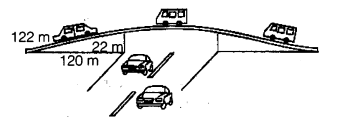Solution:
The lengths of the sides of the triangular walls are 122 m, 22 m and 120 m.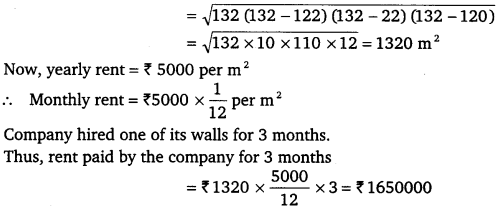Question 3.
There is a slide in a park. One of its side walls has been painted in some colour with a message ‘KEEP THE PARK GREEN AND CLEAN” (see figure). If the sides of the wall are 15m, 11 m and 6m, find the area painted in colour.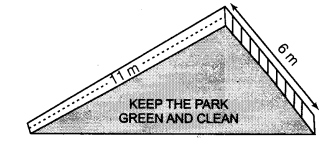Solution: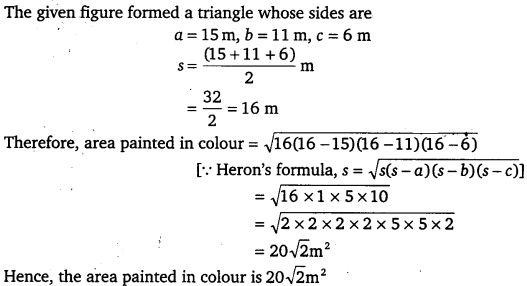Question 4.
Find the area of a triangle two sides of which are 18 cm and 10 cm and the perimeter is 42 cm.

Solution:
Let the sides of a triangle, a = 18 cm, b = 10 cm and c
We have, perimeter = 42 cm
⇒  a + b + c = 42
⇒ 18 +10 + c = 42
⇒  c = (42 28) cm
⇒ c = 14 cm
Now a + b + c$s=\cfrac { a+b+c }{ 2 }$Question 5.
Sides of a triangle are in the ratio of 12:17: 25 and its perimeter is 540 cm. Find its area.

Solution:
Given, sides of a triangle are in the ratio 12 :17 :25 and perimeter = 540 cm .
Let the sides of a triangle be a a = 12x, b = 17x and c = 25x.
Perimeter of a triangle = a + b + c
540 = 12x + 17x + 25x
⇒ 540 = 54x
⇒ x =$\cfrac { 540 }{ 54 }$
∴ x = 10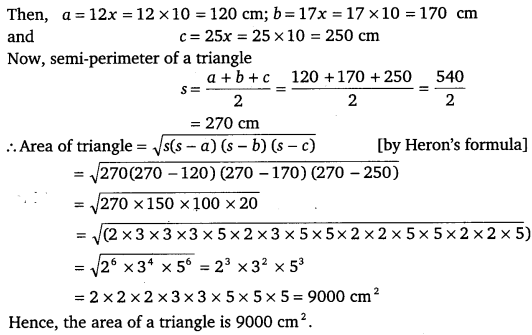Question 6.
An isosceles triangle has perimeter 30 cm and each of the equal sides is 12 cm. Find the area of the triangle.

Solution:
Here, each equal side of an isosceles triangle is 12 cm.
And perimeter of the given triangle is 30 cm
∴ Third side of the triangle = 30 -12 -12 = 6 cm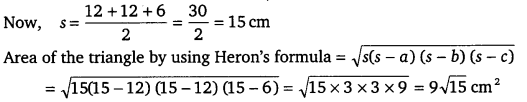#### CLASS 9 MATH NCERT SOLUTION FOR CHAPTER – 12 HERON’S FORMULA EX – 12.2

Lorem ipsum dolor sit amet, consectetur adipiscing elit. Phasellus cursus rutrum est nec suscipit. Ut et ultrices nisi. Vivamus id nisl ligula. Nulla sed iaculis ipsum.

Company Name